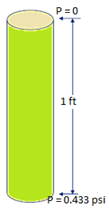### Pressure and depth:

“Pressure and Depth” is the FUNDAMENTAL relationship in the oil industry. Your understanding of the concept is crucial. The easiest way to calculate pressure from depth is to use the pressure gradient of the given fluid.

Pressure gradients for incompressible fluids have units of pressure/depth. For example, psi/ft, bar/m.

Pressure gradient seems difficult, but it is simply using the density of the fluid and converting units:

The density of pure water is 1000 kg/m3. To convert to gradient:• 1 kg = 2.2 pounds
• 1 m = 39.37 inches
• 1 m = 3.28 feet0.433 is the gradient for pure water (SG = 1) in Imperial units, remember it.

NB: Specific Gravity is always relative to pure water.